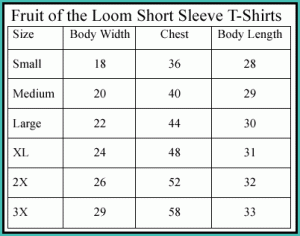# Measurements Charts

This measurement chart shows the differencesand equivalents of customary US measurements and those used in the metric system. You can use this as reference when comparing figures from different sources.

### US Measurements: Length

1 foot is equal to 1 inch, while 1 yard (yd) is equal to 36 in. 1 yd is equal to 3 feet. A baseball bat is about a yard long, for example. 1 mile (mi) is equal to 63,360 in. In terms of feet, 1 mi is equal to 5280 ft. That is also 1760 yd.

### Metric System: Length

1 cm=10 mm. A good example is a paperclip; it is a centimeter wide. 1 decimeter (dm) is 100 mm or 10 cm. This is the length of an average sized crayon. As any measurement chart will show, a meter (m) is equal to 1000 mm or 100 cm or 10 m. 1 kilometer (km) is equal to 1,000,000 mm or 1,000,000 mm. It is also equal to 0,000 dm and 1000 m.

### US Measurements: Weight

1 pound (lb) is equal to 16 oz. This is the typical weight of a loaf of bread. A single bread slice weighs about an ounce. 1 ton (T) = 32,000 oz or about 2000 lb. The average family sized car weighs a ton.

### Metric System: Weight

A gram is about 0.035 ounce. A packet of sugar tips the scale at one gram. A measurement chart will also show that 1000 grams is equal to 1 kilogram. 1000 kilograms is equal to 1 ton.

### US Measurements: Capacity

A pint is equal to about 2 cups. A tall glass of lemonade or small soup bowl holds a pint. 1 quart (qt) is equal to 4 cups. A quart is also equal to two pints. Oil in America comes in a quart sized package. A gallon (gal) is equal to 16 cups. A gallon is also equivalent to 8 pints or 4 quarts. In the US, large milk containers are sold in gallons.

### Metric System: Capacity

A metric measurement chart will show that 1 liter is equal to 1000 milliliters. A kiloliter is equal to 1.31 cubic pints whole a dekaliter is equal to 10 liters.

### Food Measurements

1 tablespoon is equal to 3 teaspoons. 1/16 cup is equal to 1 tablespoon. 1/8 cup is the same as 2 tablespoons, while 1/6 cup is equal to 2 tablespoons plus 2 teaspoons.

1/4 cup is equivalent to 4 tablespoons. 1/3 cup is equivalent to 5 tablespoons plus a teaspoon. 3/8 cup is equal to 6 tablespoons and 1/2 cup is the same as 8 tablespoons.

2/3 cup is equal to 10 tablespoons plus 2 teaspoons. 3/4 cup is equal to 12 tablespoons. 1 cup is the same as 48 teaspoons or 16 tablespoons. 8 fluid ounces (fl oz) is equivalent to 1 cup. 16 ounces (oz) is the same as 1 pound.

Because the US and the world measuring standards are different, using this measurement chart can be handy. It can prevent a lot of confusion and mistakes.